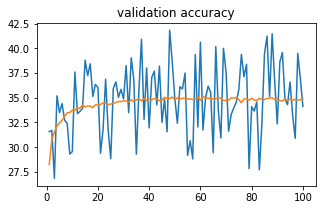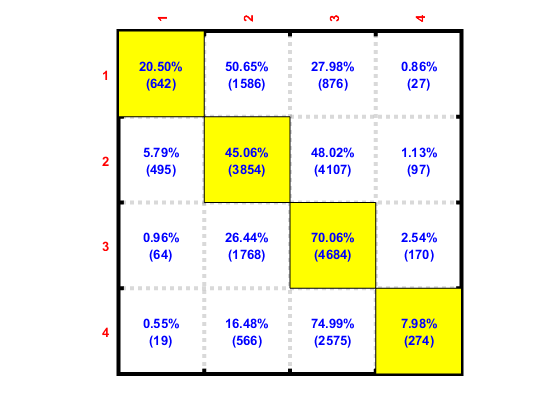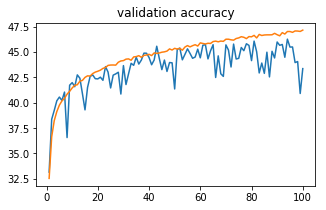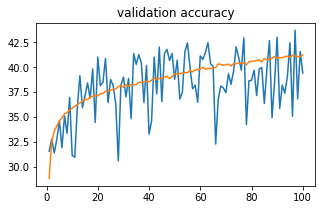# Weighted sampling & Weighted CE loss not helping

I am addressing a 4 class classification problem. It’s a 1D data set with ~145000 samples and 70 features. MLP architecture: [70 - 400 - 4]. Adam with 10^-5 learning rate. batch size=32. Currently I’m getting train accuracy of ~55% and validation accuracy of ~52-53%. I was hoping addressing class imbalance would improve network performance.

I tried the following to overcome class imbalance problems.

Try 1: Weighted sampling

u = np.unique(labels_t)
w = np.histogram(labels_t, bins=np.arange(min(u), max(u)+2))
weights = 1/torch.Tensor(w)
sampler = torch.utils.data.sampler.WeightedRandomSampler(weights.double(), batch_size)

train_data = torch.utils.data.TensorDataset(features_t,labels_t)

val_data = torch.utils.data.TensorDataset(features_v,labels_v)

Try 2: Weighted Loss

u = np.unique(labels_t)
w = np.histogram(labels_t, bins=np.arange(min(u), max(u)+2))
weights = 1/torch.Tensor(w)

loss = F.nll_loss(output, target, weight=weights)

^changed both in train function and validation function

Neither of these seems to give improvements over simply training without addressing the issue of class imbalance. Is there anything I’m overlooking?

Could you post your confusion matrix?
Is the model ignoring the minority classes?

A minor side note: Instead of `np.unique` and `np.histogram` you can directly get the class counts with:

``````_, counts = np.unique(labels_t, return_counts=True)
``````
2 Likes

I’m retraining my model to get confusion matrix. Does the following plots provide any insight into where I might be going wrong?

Orange curve is train accuracy and blue curve is validation accuracyFig1: training without addressing class imbalance. N/w arch: [70-400-4]Fig2: training with weighted loss (Try 2). N/w arch: [70-400-4]

In the later case, training accuracy seems to saturate. Also, what could be the reason behind fluctuation in validation accuracy in the 2nd plot.

Thanks!

How many classes do you have and how is the imbalance proportion wise?
The accuracies in the first figure might come from overfitting to the majority class, thus the general accuracy seems to be good, while the mean per class accuracies are bad.
Have a look at the Accuracy paradox to see, why the accuracy might lead to wrong assumptions in an imbalanced setting.

I’ll wait for the confusion matrices before further speculation.1 Like

It’s a 4 class classification.
The number of samples for the classes in train set look something like: [15%, 40%, 30%, 15%]
Validation set is similar.

Orange curve is train accuracy and blue curve is validation accuracy

MLP without weighted LossMLP with weighted LossOk, the diagonal numbers in the matrix have increased using weighted loss – which is a good sign.Any inputs on how I can improve train and validation accuracy? Unfortunately, I don’t have the privilege of getting more train data. I’m training a MLP: [70 6000 4] without weighted loss, just to see if I’m able to overfit on train data.

Do let me know your thoughts. Thank you!

1 Like

Well, I would switch to mean per class accuracy as my metric which I would like to improve. Depending on your use case there might be a better metric, but in my opinion it’s better for an imbalanced dataset than the general accuracy.
I assume the rows of your confusion matrix are your predictions and the columns the ground truth? If so, you can just divide the diagonal by the sum over the columns to get the per class accuracies. Then just calculate the mean and you’ll have a metric to optimize.

Overfitting on a small dataset is always a good starter to see, if your architecture is suitable.

What are the results using the `WeightedRandomSampler`? I think it should also yield good results. However, since the minority classes are oversampled using the default settings, your model might overfit to these. Are you able to apply some data augmentation on your input? Maybe a small amount of random noise on the features?

Rows are ground truths and cols are predictions. The mean per class accuracy metric you suggested would be in range 0-1. Do I use MSE loss layer, with 1 as the target always?

Something like this,

d = np.diag(confMat)
s = np.sum(confMat,1)
l = np.mean(d/(s+1)) #(s+1) to avoid 0 in denominator
loss = F.MSELoss(l, 1)

Would this help improve per class accuracy?

Nope. I quickly tried SMOTE from sklearn. Haven’t explored the results yet!

A question regarding ‘weight’ parameter passed to nll_loss. My priority is to get class 4 right (always), then class 3… so on. So can I pass in weight=[0.1, 0.2, 0.3, 0.4], without taking into consideration the number of samples present in each class? Will this ensure that the network get class 4 right (as best as possible)?

Thanks!

I doubt you can use the mean per class accuracy to train your model. It’s just a metric to see, which model performs better. You can also use it for early stopping etc.

Your percentage doen’t add up to 100%. How did you calculate it?
It won’t ensure the network will get class3 right, but it’ll guide the model to focus stronger on the class.

So to make sure I got you right, you suggest that I train model using wighted NLL_Loss but pick model based on best mean per class accuracy?

Where exactly?

This would be one possible approach, which is better in my opinion than the “global” accuracy.
If you want to focus on a specific class, you should change your metric of course.
Did you get any good results using SMOTE?

Hahaha, nevermind. I’ve looked wrong (or was a bit stupid).

SMOTE didn’t help. I think the problem is, the features are handcrafted - some of them are discrete values. Interpolating with SMOTE helps model learn faster however it does not do well on validation set.

On the other hand, batch norm helped a bit. Got a 4% increase in accuracy. Would you recommend batch norm+l2 regularization or batch norm+dropout?

4% increase sounds good! I usually try Dropout first and then weight decay. But I don’t have an idea which one would work for your use case.

What kind of features do you have?
Are you normalizing the features?

They are 1D features - most of them are FC layer values from another CNN, some of them discrete (I don’t know what they mean).

I just add batch norm layer after data layer.
Data => BN1 => FC1 => BN2 => ReLU => FC2 => Log Softmax

Is this CNN pre-trained or are you learning it end to end?
What is the input of the CNN?
How come they are discrete? Did you apply some operation on the fc features?

Its a MLP that I’m training. You can think of this as an MLP in pipeline after a CNN

I don’t know from where those discrete values are obtained. And they are surely not obtained by applying some operation on FC features.

Could you check the ranges of your features?
If there are some discrete features, they might be in a completely other numerical range, which might be problematic for the training.

This is what my feature range looks like

feature number, min value, max value

For example, 8th feature ranges from 1 to 4

Since the range are quite different, some are in `[0, 1]`, while other are in `[0, 90000]`, you should consider using a normalization technique for your input data.
Have a look at the StandardScaler from scikit-learn.
Don’t forget to fit it on the training data and just transform the test data.Oh yes! I tried this. Wouldn’t batch norm after data layer have similar effect?